Maths-
General
Easy

Question

# 12 men can dig a pond in 8 days. How many men can dig it in 6 days?Hint:

## The correct answer is: 6 days.

### Let the number of days be represented as x and the number of men be represented as y. The number of men will increase if the number of days are decreased so we can conclude that y is in inverse relationship with x. Let’s say the proportional relationship is given asy =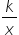……..(1)Step 1 of 2:It is given that 12 men can dig a pond in 8 days i.e. x = 8 and y = 12. Putting the values in equation (1)12 =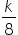k = 12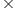8  = 96Step 2 of 2:Now we are asked to find the number of men if 6 days are taken by them. Let the number of men be “m”. So, x = 6 and y = m. Putting the values in equation (1)m =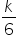Now, put the value of k = 96m =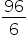m = 16 menFinal Answer: Hence, 16 men can dig the pond in 6 days.#### With Turito Foundation.#### Get an Expert Advice From Turito.